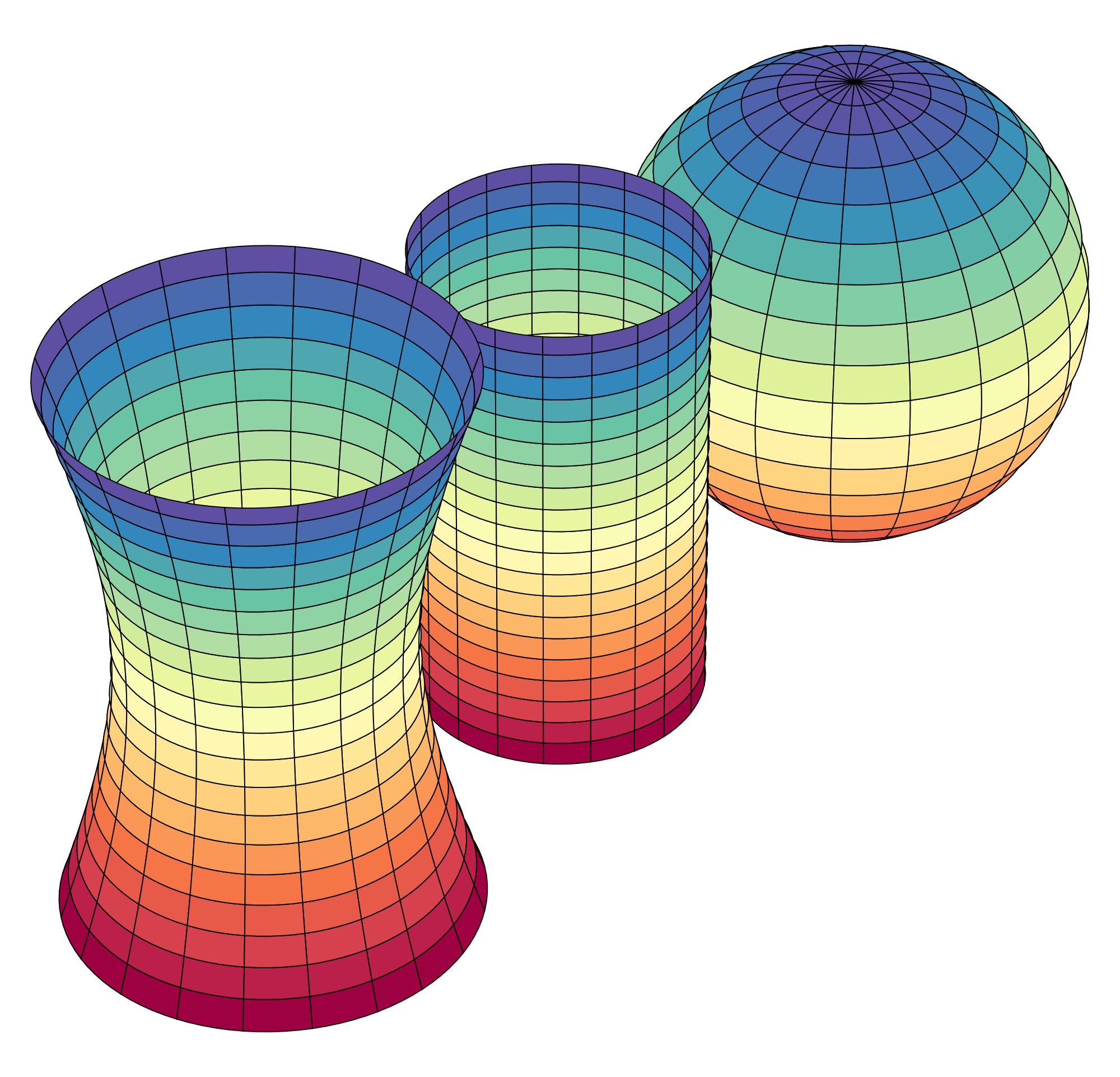# Online Elementary Linear Algebra Course - Distance Calculus

What is the difference between "Linear Algebra" and "Elementary Linear Algebra"?

####Upper Division Math Courses

When you see the term "Elementary" in the title, they are specifically talking about the sophomore-level Linear Algebra course commonly taken after Calculus II.

There are more advanced Linear Algebra courses available, usually taken by math and computer science students, which are sometimes called Advanced Linear Algebra, or Computational Linear Algebra. These are courses that jump into the math theory of Linear Algebra, and usuall require a student have had a course in group theory or abstract algebra.

Most students looking for a Linear Algebra course are looing for "Elementary Linear Algebra".

What is the difference in a taking a textbook/lecture based course on linear algebra vs Distance Calculus Linear Algebra?

There are huge differences between our Linear Algebra course, which is Mathematica-based, and a traditional textbook/lecture course.

Our Mathematica-based course is highly experimentation-based - running experiments graphically, numerically, algebraically, to get at the concepts of linear algebra as you would in a chemistry or physics lab.

Traditional Linear Algebra courses usually center on doing small calculations you can do by hand, and then concentrating on abstract concepts of linear algebra, avoiding difficult calculations in favor of ... sometimes proofs, sometimes just calculations that can be completed by humans on paper.

Our Distance Calculus Linear Algebra course is perfect for students who might be interested in a "Computational Linear Algebra" course, although that course title is usually reserved for courses where the students are actually trying to learn how to program software like Mathematica or manual programs to do the computations. Those courses are more about numerical analysis, as they assume you already completed an Elementary Linear Algebra course before.

Here is a video about our Linear Algebra course via Distance Calculus @ Roger Williams University:

## After AP Calculus for High School Students

Linear Algebra course can best be described as a "first course in the study of Linear Algebra and Matrix Theory".

This course has many names, all being equivalent:
• Linear Algebra
• Matrix Theory
• Linear Systems of Equations
• Linear Spaces

Our Linear Algebra course differs from a classroom/textbook-based course in that we employ Mastery Learning so that you complete all assignments at 100% to assure competancy, as well as our curriculum shifting the course to a laboratory-style course, where theorem/lemma/proof type exposition is replaced by running experiments in Mathematica as you would in a science laboratory to empirically deduce the concepts and behaviors of Linear Algebra, both solvable (classically) via hand-based techiques, as well as studying Linear Algebra that can only be solved and investigated graphically and numerically using a computer. The Linear Algebra curriculum is highly visual and based upon observations of experiments run in Mathematica.

At Distance Calculus, we call our "Linear Algebra" course as Linear Algebra - DMAT 335 - 3 credits.

Below are some links for further information about the Linear Algebra course via Distance Calculus @ Roger Williams University.

## Distance Calculus - Student ReviewsDate Posted: Aug 23, 2020
Review by: Sean Metzger
Student Email: seanmetzger78@gmail.com
Courses Completed: Differential Equations
Review: A lifesaver. When I found out I needed a course done in the last weeks of summer I thought there was no way i'd find one available, but this let me complete the course as quickly as I needed to while still mastering the topics. Professor always got back to me very quickly and got my assignments back to me the next day or day of. Can't recommend this course enough for students in a hurry or who just want to learn at their own pace.
Transferred Credits to: Missouri University of Science and TechnologyDate Posted: Feb 28, 2020
Review by: Karen N.
Courses Completed: Calculus I, Calculus II
Review: Awesome classes! I was really weak with Calculus, so I retook Calc 1 and kept going into Calc 2. I feel like I finally understood Calculus. The finals were pretty thorough, but not nearly as stressful as the blue book exams. I highly recommend these courses!
Transferred Credits to: VariousDate Posted: Jan 12, 2020
Review by:
Courses Completed: Calculus I, Calculus II
Review: I needed to brush up on my high school calculus and finally take Calc II before starting a graduate program that needed them as prereqs. This was perfect choice to fit in that summer. Got done at fast pace that I wanted and needed. Also had added bonus of one on one feedback and help when needed. Video lessons were better than many on campus instructors in large lecture settings. Recommend for anyone needing to satisfy prereqs at home institution.
Transferred Credits to: University of Michigan